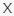September 23, 2023
Latest:
Videofacts

# Maths Quiz VideoFacts

Q 21. Do you know the answer to this one?

Choices:

2
4
6
8

8

Q 22. Do you know the answer to this question?

Choices:

2650
2640
2600
2700

2600

Q 23. Can you answer this question?

Choices:

0.3
0.03
0.003
300

0.03

Q 24. What is the answer here?

Choices:

30
-90
-30
90

30

Q 25. How many triangles can you count?

Choices:

10
12
14
16

14

Q 26. What does ‘X’ equal here?

Choices:

53
57
63
67

63

Q 27. What is ‘Y’ equivalent to?

Choices:

5
6
7
8

5

Q 28. Tell us which digits have been replaced by question marks in this sum.

Choices:

5, 6
8, 9
6, 7
4, 5

6, 7

Q 29. Calculate the correct answer to this equation.

Choices:

83.6
81.5
91.3
87.1

83.6

Q 30. Do you know the answer to this conundrum?

Choices:

16
18
20
22